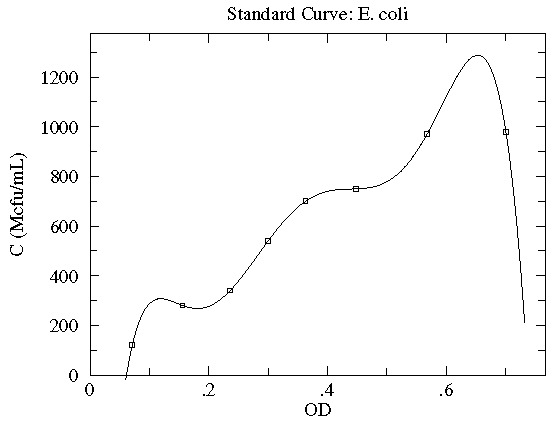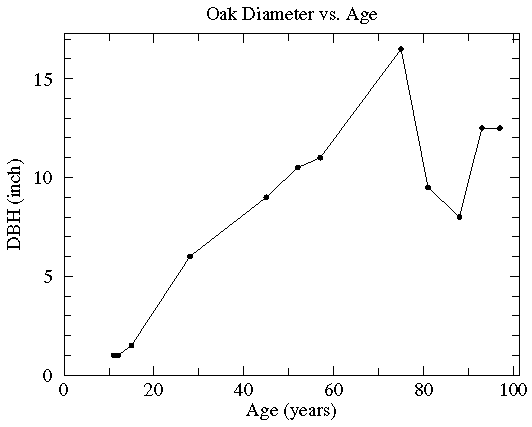## WAPP+: Web-based Analysis Program for Physics

This page is intended to provide a unified start to determining a "trendline" for a dataset {(xi,yi)}. If you're new to this page you proably want to carefully read the following discussion of the fitting options, but if you're experienced this process before you may just want check that you satisfy the conditions recorded in the summary and then click to start entering your data. What follows is some examples (from other pages here) of finding trendlines.

If you just want to plot data, try this: No trendline fit, just plot.

### Summary

In order to do find a trendline you need:

• Several (say N) pairs of related data: (xi , yi ); i=1,2,...,N. The choice of which variable is x and which is y can be made on several bases:
• Which has the least error?
• Which is the controlling variable?
• Which do you intend to calculate in the future using the generated formula?
• What did your instructor tell you to do? (Note: if you've been told to plot: "A vs. B", B is on the x-axis.)

• Ideally you should have an estimate of the accuracy of the x and y values of the data: the so called x and y-errors (xei and yei ). The accuracy estimate may be a general rule (e.g., all the y-values are accurate to 3%) or individual estimates for each datapoint. The first thing you will be asked is what sort of errors you have. Making good estimates of errors is perhaps the most difficult part of doing science, however it is not a topic I've written on here: ask your instructor when in doubt!

• Some idea of an appropriate type of curve to fit. If theory and instructors have provided no hints, start with a linear fit. If x or y data spans more than a factor of 10, consider log transformations of that variable. If the curve is "nearly" linear, consider a quadratic.

• An understanding the nature of the parameter estimates (and the reported uncertainty in those parameters) provided by this site and the ability to figure out the units of those parameter estimates.

• What is the current form of your data? Are the numbers already available on this computer (for example in a spreadsheet)? In this case you can probably just copy and paste that data into one of our bulk-entry forms. If your data consist of numbers on a sheet of paper you'll be probably better off using "pointwise" data entry -- in which case I'll want to know how many datapoints you have (i.e., N). In either case, this web page limits the number of datapoints to N<100. Your errors may be in the form of a formula or a list of numbers.

### y errors?

No y errors
Enter a formula (or a constant) for y errors
Enter y error for each data point

### x errors?

No x errors
Enter a formula (or a constant) for x errors
Enter x error for each data point

### pointwise data entry

Number of data points:

# A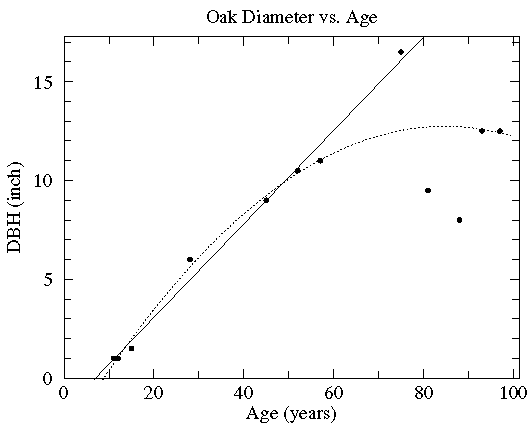In this case the diameter of various oak trees is plotted as a function of the tree's age and two possible "trendlines" are displayed: a straight line and a quadratic curve...we mean to include various curves in our definition of "trendline". The commonly used process of determining these trendlines is called least squares fitting or regression. There are other less common options which are described here. While every measuring device has limited precision (here tree diameter measured with a tape measure and tree age measured by counting tree rings), these measurement errors are not the source of the variation in this data (instead local growth environment and genes are the likely source of the variation). Since the extent of the variation in (x,y) is unknown, we lack the usual x and y errors.

# B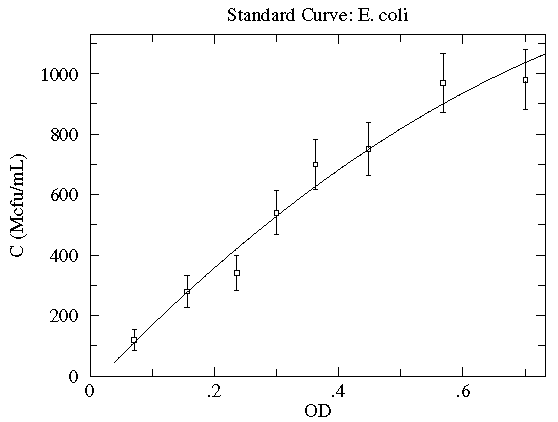In this case the concentration of E. coli bacteria is plotted as a function of the optical depth (read: cloudiness) of the corresponding suspension. Here, in addition to the datapoints, we have an estimate of the likely variation in the y quantity. These "y-errors" (yei) are displayed as vertical error bars around each data point. Notice the curve entirely misses one error bar, and nearly misses a couple of other error bars. This is entirely expected; in fact, typically a good trendline will miss (but not by a lot) 1/3 of the error bars. [As noted elsewhere, there is no universal choice for the size of an error bar which makes this statement a bit problematic.] There is of course uncertainty in each measurement of the x quantity (optical depth as recorded on a Spectronic 20D's digital meter), but it was deemed "negligible" compared to the variation in the y quantity. [In fact the error in OD is mostly systematic and not of great relevance as long as the same equipment is always used for the measurement.] The trendline here is a quadratic:

y = A + Bx + Cx2

Very simplified theory (e.g., Beer-Lambert Law) might suggest an approximately linear relationship, and here we find a small, negative value of C improves the fit. Do notice that this fit curve would provide crazy results if applied beyond the range of the data...for example negative concentrations if OD>2.5. By displaying the fit curve along with the data we can understand the reliability of the curve within the range of the data (this is basically interpolation). How well the curve works beyond the range of the data (basically extrapolation) is at best a guess.

# CIn this case the current flowing through a vacuum tube is plotted as a function of the filament temperature. Here the likely variation in both x (xei) and y (yei) are displayed as error bars. While the trendline looks like a line, a glance at the axes shows logarithmic (y) and inverse (x) scaling. In fact the trendline is an Arrhenius curve:

y = A exp(B/x)

Also notice something that should strike you as odd: the trendline goes pretty much dead center through each datapoint. The expected level of variation did not occur. While this might be a series of unlikely bull's-eyes or a blunder in determining the expected level of variation, in fact it is an example of systematic error: because of an uncertain calibration, the measured temperature may deviate from that recorded. The effect is not random; in the same situation the same temperature will be recorded: for example the meter may measure consistently high. This sort of systematic error is quite usual with most any modern measuring device. Often it does not matter; for example if you're interested in process control, it may not matter if the temperature is 25°C or 26°C just as long as it is reproducibly the same. (Of course, communication of how that process works will fail if the other guy's meter reads differently from yours.)

Finally let me stress that since every measurement is less-than-perfect, errors in both x and y is the usual case. Nevertheless, it is not uncommon for the error in one quantity to be "negligible" compared to the error in the other quantity. In this case, the usual procedure is to put the low-error quantity on the x-axis.

# DIn this case the current, I, flowing through a vacuum tube is plotted as a function voltage, V, across the tube. Idealized theory predicts the relationship to be: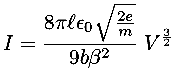and the fit looks excellent. However this data was taken with 6-digit meters; the x and y error bars are much smaller than the plotted point (box); the fitted curve turns out to be missing essentially all the error bars by many times the size of the error bars. In terms of the usual measure of quality-of-fit: reduced, this is a bad fit and idealized theory is disproved. Nevertheless, practically speaking the curve is a fair representation of the data. Idealized theory is "close" to the truth and the curve represents a very useful lie. ("Foma" to Vonnegut fans). It is common in physics to have a sequence of ever more accurate (but usually more complex) explanations [for example: the ideal gas law, van der Waals gas law, the virial expansion].

Theory in this case predicts a power law relationship:

y = A xB

While the value of A depends on variable parameters (like geometry), theory makes a definite prediction about B: B=3/2. This requirement basically comes from the dimensions of the variables: the only way the units can work out is with a particular (rational) value of B. It is fairly common for theory to require powers to be certain fixed rational values. Because of this our fitting options include fits with user specified values of B.

Incidentally for a modern silicon diode, simplified theory predicts an exponential relationship between current and voltage:

y = A exp(B x)

And while at first glance the relationship sure looks exponentiala log scale shows that the exponential relationship only holds for small V...another example of "approximate truth"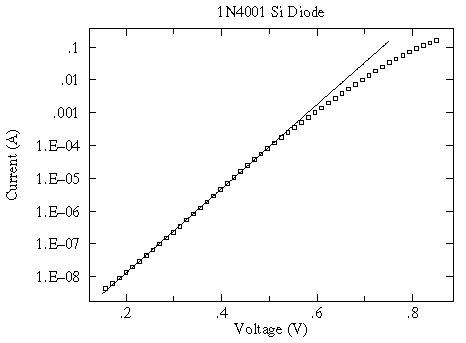Finding useful (if only approximate) relationships is common in science and engineering. It is helpful to have a name for these "approximate truth" laws; I call them "spherical cow" laws after an old joke about theoretical physicists.